Partial Differential Equations Commons™

599 Full-Text Articles 515 Authors 110,709 Downloads68 Institutions

All Articles in Partial Differential Equations

599 full-text articles. Page 1 of 22.

Analyzing Student Loan Debt Using Seir Compartmental Model Of Epidemiology, 2019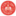George Mason University

Analyzing Student Loan Debt Using Seir Compartmental Model Of Epidemiology, Kavya Ravishankar, Dr. Padmanabhan Seshaiyer

Annual Symposium on Biomathematics and Ecology: Education and Research

No abstract provided.

Sperm Motility In Groups, 2019California State University Maritime Academy

Sperm Motility In Groups, Julie Simons

Annual Symposium on Biomathematics and Ecology: Education and Research

No abstract provided.

Multidisciplinary Education And Research In Biomathematics For Solving Global Challenges, 2019George Mason University

Multidisciplinary Education And Research In Biomathematics For Solving Global Challenges, Padmanabhan Seshaiyer

Annual Symposium on Biomathematics and Ecology: Education and Research

No abstract provided.

2019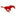Southern Methodist University

Parallel Multipole Expansion Algorithms And Their Biology Applications, Jiahui Chen

Mathematics Theses and Dissertations

N-body pairwise interactions are ubiquitous in scientific areas such as astrophysics, fluids mechanics, electrical engineering, molecular biology, etc. Computing these interactions using direct sum of an O(N) cost is expensive, whereas multipole expansion methods, such as the fast multipole method (FMM) or treecode, can reduce the cost to O(N) or O(N log N). This thesis focuses on developing numerical algorithms of Cartesian FMM and treecode, as well as using these algorithms to directly or implicitly solve biological problems involving pairwise interactions. This thesis consists of the following topics. 1) A cyclic parallel scheme is developed to handle ...

Smoothness Of Defining Functions And The Diederich-Fornæss Index, 2019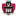University of Arkansas, Fayetteville

Smoothness Of Defining Functions And The Diederich-Fornæss Index, Felita Nadia Humes

Theses and Dissertations

Let Ω ⊂ Cn be a smooth, bounded, pseudoconvex domain, and let M ⊂ ∂Ω be a complex submanifold with rectifiable boundary. In 2017, Harrington studied the equation dM A = α ̃ on M, where α ̃ is D’Angelo’s 1-form and A is real. In this thesis, we will study a non-pseudoconvex example in which M has a non-rectifiable boundary. In spite of the lack of topological obstructions on the boundary, there are no continuous solutions to dM A = α ̃.

2019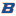Boise State University

Radial Basis Function Finite Difference Approximations Of The Laplace-Beltrami Operator, Sage Byron Shaw

Boise State University Theses and Dissertations

Partial differential equations (PDEs) are used throughout science and engineering for modeling various phenomena. Solutions to PDEs cannot generally be represented analytically, and therefore must be approximated using numerical techniques; this is especially true for geometrically complex domains. Radial basis function generated finite differences (RBF-FD) is a recently developed mesh-free method for numerically solving PDEs that is robust, accurate, computationally efficient, and geometrically flexible. In the past seven years, RBF-FD methods have been developed for solving PDEs on surfaces, which have applications in biology, chemistry, geophysics, and computer graphics. These methods are advantageous, as they are mesh-free, operate on arbitrary ...

2019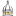The University of Southern Mississippi

Krylov Subspace Spectral Methods With Non-Homogenous Boundary Conditions, Abbie Hendley

Master's Theses

For this thesis, Krylov Subspace Spectral (KSS) methods, developed by Dr. James Lambers, will be used to solve a one-dimensional, heat equation with non-homogenous boundary conditions. While current methods such as Finite Difference are able to carry out these computations efficiently, their accuracy and scalability can be improved. We will solve the heat equation in one-dimension with two cases to observe the behaviors of the errors using KSS methods. The first case will implement KSS methods with trigonometric initial conditions, then another case where the initial conditions are polynomial functions. We will also look at both the time-independent and time-dependent ...

The Martingale Approach To Financial Mathematics, 2019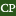California Polytechnic State University, San Luis Obispo

The Martingale Approach To Financial Mathematics, Jordan M. Rowley

Master's Theses and Project Reports

In this thesis, we will develop the fundamental properties of financial mathematics, with a focus on establishing meaningful connections between martingale theory, stochastic calculus, and measure-theoretic probability. We first consider a simple binomial model in discrete time, and assume the impossibility of earning a riskless profit, known as arbitrage. Under this no-arbitrage assumption alone, we stumble upon a strange new probability measure Q, according to which every risky asset is expected to grow as though it were a bond. As it turns out, this measure Q also gives the arbitrage-free pricing formula for every asset on our market. In considering ...

2019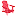University of Lynchburg

Mathematical Models: The Lanchester Equations And The Zombie Apocalypse, Hailey Bauer

Undergraduate Theses and Capstone Projects

This research study used mathematical models to analyze and depicted specific battle situations and the outcomes of the zombie apocalypse. The original models that predicted warfare were the Lanchester models, while the zombie apocalypse models were fictional expansions upon mathematical models used to examine infectious diseases. In this paper, I analyzed and compared different mathematical models by examining each model’s set of assumptions and the impact of the change in variables on the population classes. The purpose of this study was to understand the basics of the discrete dynamical systems and to determine the similarities between imaginary and realistic ...

Underground Storage Tank Impact Model, 2019Carroll College

Underground Storage Tank Impact Model, Amy Telck

Carroll College Student Undergraduate Research Festival

Leaking underground storage tanks (UST) pose a threat to the surround- ing environment and population. The Montana Department of Environmental Quality has completed two phases of a risk analysis with the purpose of identifying the USTs at the greatest risk of leaking in order to reallocate department resources. This analysis builds upon prior analyses in three ways: (1) identify how UST upgrades can reduce the risk of UST leaking, (2) how UST characteristics and upgrades relate to the cost of remediation, and (3) estimating the environmental impact of a release.

2019University of Lynchburg

Mathematical Modeling: Lanchester Equations And The Zombie Apocalypse, Hailey Bauer

Student Scholar Showcase

Mathematical models are systems using mathematical concepts to explain various problems. Mathematical models examine realistic issues such as the different outcomes of wars and fictional problems such as the upcoming zombie apocalypse. The original predictor models of warfare were the Lanchester models, while the zombie apocalypse is a fictional expansion upon mathematical models for infectious diseases. In this paper, we examined and compared the basic versions of these dynamic models by analyzing each model’s set of variables, assumptions, and objectives. The basic versions of the Lanchester equations are the area aimed model and the aimed fire model. While the ...

2019The University of Southern Mississippi

Enhancement Of Krylov Subspace Spectral Methods Through The Use Of The Residual, Haley Dozier

Dissertations

Depending on the type of equation, finding the solution of a time-dependent partial differential equation can be quite challenging. Although modern time-stepping methods for solving these equations have become more accurate for a small number of grid points, in a lot of cases the scalability of those methods leaves much to be desired. That is, unless the timestep is chosen to be sufficiently small, the computed solutions might exhibit unreasonable behavior with large input sizes. Therefore, to improve accuracy as the number of grid points increases, the time-steps must be chosen to be even smaller to reach a reasonable solution ...

Dispersive Hydrodynamics In Viscous Fluid Conduits, 2019University of Colorado, Boulder

Dispersive Hydrodynamics In Viscous Fluid Conduits, Michelle Maiden

Applied Mathematics Graduate Theses & Dissertations

Viscous fluid conduits provide an ideal system for the study of dissipationless, dispersive hydrodynamics. A dense, viscous fluid serves as the background medium through which a lighter, less viscous fluid buoyantly rises. If the interior fluid is continuously injected, a deformable pipe forms. The long wave interfacial dynamics are well-described by a dispersive nonlinear partial differential equation called the conduit equation.

Experiments, numerics, and asymptotics of the viscous fluid conduit system will be presented. Structures at multiple length scales are characterized, including solitary waves, periodic waves, and dispersive shock waves. A more generic class of large-scale disturbances is also studied ...

2019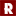University of Mary Washington

Algorithms To Approximate Solutions Of Poisson's Equation In Three Dimensions, Ray Dambrose

Rose-Hulman Undergraduate Mathematics Journal

The focus of this research was to develop numerical algorithms to approximate solutions of Poisson's equation in three dimensional rectangular prism domains. Numerical analysis of partial differential equations is vital to understanding and modeling these complex problems. Poisson's equation can be approximated with a finite difference approximation. A system of equations can be formed that gives solutions at internal points of the domain. A computer program was developed to solve this system with inputs such as boundary conditions and a nonhomogenous source function. Approximate solutions are compared with exact solutions to prove their accuracy. The program is tested ...

Analytical Wave Solutions Of The Space Time Fractional Modified Regularized Long Wave Equation Involving The Conformable Fractional Derivative, 2019Jessore University of Science and Technology

Analytical Wave Solutions Of The Space Time Fractional Modified Regularized Long Wave Equation Involving The Conformable Fractional Derivative, M. Hafiz Uddin, Md. Ashrafuzzaman Khan, M. Ali Akbar, Md. Abdul Haque

Karbala International Journal of Modern Science

The space time fractional modified regularized long wave equation is a model equation to the gravitational water waves in the long-wave occupancy, shallow waters waves in coastal seas, the hydro-magnetic waves in cold plasma, the phonetic waves in dissident quartz and phonetic gravitational waves in contractible liquids. In nonlinear science and engineering, the mentioned equation is applied to analyze the one way tract of long waves in seas and harbors. In this study, the closed form traveling wave solutions to the above equation are evaluated due to conformable fractional derivatives through double (G'⁄G,1⁄G)-expansion method and the ...

Finding Positive Solutions Of Boundary Value Dynamic Equations On Time Scale, 2019Selected Works

Finding Positive Solutions Of Boundary Value Dynamic Equations On Time Scale, Olusegun Michael Otunuga

Olusegun Michael Otunuga

This thesis is on the study of dynamic equations on time scale. Most often, the derivatives and anti-derivatives of functions are taken on the domain of real numbers, which cannot be used to solve some models like insect populations that are continuous while in season and then follow a difference scheme with variable step-size. They die out in winter, while the eggs are incubating or dormant; and then they hatch in a new season, giving rise to a non overlapping population. The general idea of my thesis is to find the conditions for having a positive solution of any boundary ...

2019University of Colorado at Boulder

Strong Form Meshfree Collocation Method For Higher Order And Nonlinear Pdes In Engineering Applications, Andrew Christian Beel

Civil Engineering Graduate Theses & Dissertations

The strong form meshfree collocation method based on Taylor approximation and moving least squares is an alternative to finite element methods for solving partial differential equations in engineering applications. This study examines how the proposed alternative method solves (i) higher-order and (ii) nonlinear partial differential equations. First, the proposed method is formulated in Chapter 2 for the general discretization and solution of strong forms of partial differential equations. Chapter 3 presents the convergence and error behavior of the proposed method for the fourth-order Stommel-Munk equation for wind-driven ocean circulation, as well as the numerical solution of this equation on a ...

2019Iowa State University

Long-Run Average Cost Minimization Of A Stochastic Processing System, Bowen Xie

Creative Components

A long-run average cost problem in stochastic control theory is addressed. This problem is related to the optimal control of a production-inventory system which is subjected to random fluctuation. The approach taken here is based on finding a smooth solution to the corresponding Hamilton-Jacobi-Bellman equation. This solution in turn is used to derive an optimal process for the above long-run average cost problem. Using the invariant distributions for positive recurrent diffusion processes, another derivation for the optimal long-run average cost is provided here.

2019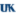University of Kentucky

Approximations In Reconstructing Discontinuous Conductivities In The Calderón Problem, George H. Lytle

Theses and Dissertations--Mathematics

In 2014, Astala, Päivärinta, Reyes, and Siltanen conducted numerical experiments reconstructing a piecewise continuous conductivity. The algorithm of the shortcut method is based on the reconstruction algorithm due to Nachman, which assumes a priori that the conductivity is Hölder continuous. In this dissertation, we prove that, in the presence of infinite-precision data, this shortcut procedure accurately recovers the scattering transform of an essentially bounded conductivity, provided it is constant in a neighborhood of the boundary. In this setting, Nachman’s integral equations have a meaning and are still uniquely solvable.

To regularize the reconstruction, Astala et al. employ a high ...

Multiscale Mathematical Modelling Of Nonlinear Nanowire Resonators For Biological Applications, 2019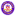Wilfrid Laurier University

Multiscale Mathematical Modelling Of Nonlinear Nanowire Resonators For Biological Applications, Rosa Fallahpourghadikolaei

Theses and Dissertations (Comprehensive)

Nanoscale systems fabricated with low-dimensional nanostructures such as carbon nanotubes, nanowires, quantum dots, and more recently graphene sheets, have fascinated researchers from different fields due to their extraordinary and unique physical properties. For example, the remarkable mechanical properties of nanoresonators empower them to have a very high resonant frequency up to the order of giga to terahertz. The ultra-high frequency of these systems attracted the attention of researchers in the area of bio-sensing with the idea to implement them for detection of tiny bio-objects. In this thesis, we originally propose and analyze a mathematical model for nonlinear vibrations of nanowire ...